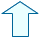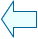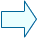EXPONENTIAL EQUATIONS AND SIMULTANEOUS EXPONENTIAL EQUATIONS Section: Algebra

1. EXPLANATION

Exponential equations are equations where one of the sides of the equation includes an exponential expression (where the base number is constant and the variable is an exponent (x, y, etc)). For example:

a) 32-x2 = 3

b) 42x+1 = (0.5)3x+5

c) 2x-1 + 2x + 2x+1 = 7

d) ex - 5e-x + 4e-3x =0.

Initially, as in any type of equation, we need to find a value of x which solves the equation.

In simple equations, this can be worked out through simple observation. For example, look at the following equation:

2x = 4, obviously x = 2 is a solution. But, the solution is not always so straight forward.

However, let's have a look at the graph of this equation.

If we draw the graph of the exponential function y = 2x and the straight line y = 4, the "x" value of the point where the two graphs intersect gives the solution of the equation.

Look at how this works in the following window.

 The solutions of the four equations which are given as examples at the beginning of this section are not found so easily.

1.- Solve the equations graphically in the same way as we did for 2x = 4. If you draw the graphs of the two functions given by the two sides of the equation then the "x" value where the two graphs intersect is the solution. Use the 'Type 1' window below to find the solution.

2.- Solve the equations graphically by making the RHS equal to 0. Draw the graph of the function which corresponds to the LHS. The points where it cuts the X-axis are the solutions.

 In order to solve these equations numerically we can divide them into two basic types, as indicated below:

2. TYPE 1

The first two examples are examples of this type:

a)  32-x2 = 3  and  b)  42x+1 = (0.5)3x+5

In both cases, unlike the other two examples, we can see that there is only one term on each side of the equation ("There are no terms added together")

Let's check the solution graphically. In the following window you can see that there are two solutions for the first equation: x = 1 and x = -1.

1.- Change the two equations in the boxes at the bottom of the window and check that there is only one solution for the second equation: x = -1.
 "Be careful when writing functions with this program. For example write (0.5)3x+5 as: 0.5^(3*x+5)"

2.- In your exercise book write down that in order to solve these equations numerically we need to make sure that both sides of the equation are expressed as the same base number raised to a power and then make the exponents equal to each other. Before doing this make sure you remember all the rules governing powers and indices.

Therefore, the second example above would be solved as follows: 42x+1 = (0.5)3x+5 ; 22(2x+1) = (1/2)3x+5; 24x+2 = 1/23x+5 ; 24x+2 = 2-(3x+5) ; 24x+2 = 2-3x-5, making the exponents equal to each other we get the equation: 4x+2 = -3x-5, which is easy to solve: 7x = -7; and finally x = -1 which we obtained earlier.

 The solution obtained by making the exponents equal to each other can also be found graphically (If you would like to see how this is done, see the unit "Solving equations graphically").

3.- Solve the following equation numerically and check that the answer is the same as that given in the graph above.

32-x2 = 3

3.1.  TYPE II . EXAMPLE c)

These are exponential equations where one of the sides of the equation includes a sum of exponential expressions which cannot be simplified. Examples c) and d) at the beginning of this section are examples of this type.

They can be solved graphically in the same way as the previous type, by drawing the graph which corresponds to each side of the equation. The window illustrates how this is done for the equation:2x-1 + 2x + 2x+1 = 7, where you can see that the solution is x = 1.

 Of course, the graph of the function for each side of the equation may not look familiar and there are often large numbers in the equation which means that the graph cannot be seen clearly. This could well be the case with the equation 2x+1 +4x+1 = 520 ,which is not an uncommon equation. In such cases you can choose to "bring everything over to the LHS of the equation", as explained above, in order to solve it.

1.- In your exercise book make a note of the following explanations about the method used to solve these equations numerically.

Solving numerically: Imagine the equation 2x-1 + 2x + 2x+1 = 7. Simplify all the exponential expressions as much as possible and make them equal to each other. In this case we get 2x, and we just need to apply the appropriate rules governing powers to get:2x/2+ 2x + 2·2x = 7 .

Once we have this we can call 2x = z , which gives us the equation z/2 + z + 2z = 7. This is a simple equation which we know how to solve (Refer to the unit on equations if necessary).

Once this equation has been solved we get z = 2, which, if put back into the z equation gives us: 2x = 2. An exponential equation of the type we have already seen and whose solution is x = 1.

 3.2 . TYPE II . EXAMPLE d) We are going to use the window below to solve equation d) from he beginning of the section. d) ex - 5e-x + 4e-3x =0. 1.- Solve the equation numerically and then write the equation you have to solve numerically in the window. You should have get the solution x = 0 and the approximate solution  x = 0.67, which is obtained numerically by solving ex = 4. Do you know how to use logarithms to work out x? If you look carefully you can see that x = ln 4. You can also use the window to find the solution. 2.- Use the window and solve the following equation numerically: e) 31-x2 = 1/27 ....... f) 5x+1 + 5x = 750..... g) 4x - 2x = 2.....  h) 9x - 2·3x+2 + 81 =0.

4.-SIMULTANEOUS EXPONENTIAL EQUATIONS
As the name suggests, these refer to equations which are solved simultaneously where one or more are exponential equations.

The methods explained above are also used to solve them numerically.

In order to solve them graphically, draw the graphs of the corresponding equations which can be written exactly as they are given in our Descartes window.

1.- Solve the following simultaneous equations numerically and check the solution in the graph.

2x - 3y-1 = 5

2x+1 + 8·3y =712

 Draw the graphs of the two equations in this window and note that the solution given is the point (5,4). Hence, the solution of the equations is x = 5; y = 4.

2.- Solve the following exponential simultaneous equations numerically and graphically (change the equations in the window above):

2x + 5y = 9

2x+2 - 5y+1 = - 9

Sol: x = 2 ; y = 1Leoncio Santos CuervoSpanish Ministry of Education. Year 2001